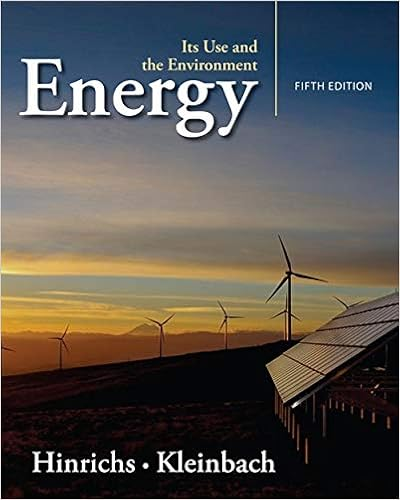# Conservation_of_Energy_at_a_Skate_Park_Final_Version_SUBMIT...

• Lab Report
• 10
• 95% (131) 124 out of 131 people found this document helpful

This preview shows page 1 - 3 out of 10 pages.

##### We have textbook solutions for you!
The document you are viewing contains questions related to this textbook.The document you are viewing contains questions related to this textbook.
Chapter 2 / Exercise 2.1
Energy: Its Use and the Environment
Hinrichs/KleinbachExpert Verified
Patrick Cody Physics Section 1 Name _____________________________________________ Class __________________ Page 1 Conservation of Energy at the Skate Park Part A: Thermal Energy Learning Objective: Describe how a change in thermal energy of the system affects the motion of molecules at the microscopic level, and the motion of the skater at the macroscopic level. PhET Friction Simulation: PhET Energy Skate Park Simulation: - basics/latest/energy-skate-park-basics_en.html Directions: 1. In the microscopic model boxes, draw and describe the motion of the skater’s molecules at her two different positions. Hint: Think about molecules in the friction simulation. 2. In the macroscopic model boxes, draw and describe the energy of the skater at the two positions .
##### We have textbook solutions for you!
The document you are viewing contains questions related to this textbook.The document you are viewing contains questions related to this textbook.
Chapter 2 / Exercise 2.1
Energy: Its Use and the Environment
Hinrichs/KleinbachExpert Verified
Name _____________________________________________ Class __________________ Page 2 Part B: Energy Changes in the Skate Park System Learning Objectives: 1. Differentiate between total energy and various forms of energy in a system. 2. Explain how each model (bar graph and pie chart) shows the total energy of the system, and draw each model for a situation with a different amounts of initial energy. 3. Describe energy changes in a system over time using both words and graphical representations. Directions: Use the simulation investigate energy in the skate park. Use different tracks on the "Introduction" and "Friction" pages. 1. Describe the system represented by the bar graph and pie chart. Explain your reasoning.
2. Investigate the meaning of “total energy”.
•••